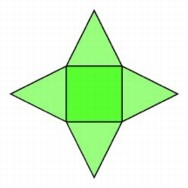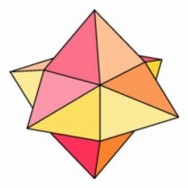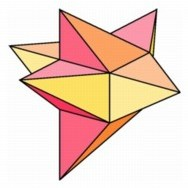Fold-Out Models of Pyramids

Numerical and algebraic patterns have led us from the familiar geometry of the plane and ordinary space to consider possible objects of higher dimensionality. But how can we begin to understand such objects if we cannot build them in our space? Fortunately the formal patterns suggest analogies in shapes as well as in formulas. By analyzing the way we can construct models of pyramids in three-dimensional space, we can see how to design analogous models for four-dimensional pyramids.The fold-out polyhedral pattern for a symmetrical cube-based hyperpyramid.The fold-out pattern for an off-center pyramid.

A cardboard model of an Egyptian pyramid can be made by attaching triangles to the sides of a square in the plane and then folding this pattern up into three-space. In the next dimension, we can, in an analogous fashion, erect a square-based pyramid on each of the six square faces of a cube. What we cannot do is fold the pattern together into a fourth dimension to construct the fourth-dimensional analogue of a square pyramid. To find the volume of the three-dimensional Egyptian pyramid that comes from the fold-out model, we can easily calculate the area of the square base, but we still have to figure out bow high the top point will be when we fold it up, and this takes some additional calculation. Similarly, we can figure out the volume of the cubical base of the folded-out four-dimensional pyramid, but it is still harder to deterinine the height in the fourth direction since we cannot actually fold the figure together.

To make a cardboard model of an off-center pyramid, which is one-third of a cube, we start with a square in the plane and at one corner we extend the two sides to obtain two edges of the same length. From tfiese we build isosceles right triangles on two sides of the square. Folding these two triangles together in three-space forms one corner of the off-center pyramid. The other two triangular faces of this pyramid are also right triangles; each has one side along an edge of the square and another side with length equal to the longest side of the isosceles triangle.The fold-out pattern for an off-center pyramid.The fold-out pattern for an off-center hyperpyramid.

An analogue of this construction in the next dimension starts with a cube. At one vertex we extend the three edges to form segments of the same length as the edges of the cube. On these edges we build three off-center pyramids identical to those constructed in the previous paragraph. If we could fold this object up into the next dimension, we would obtain an "off-center hyper-pyramid." There are also three more off-center pyramids in the boundary of this object in four-space. Four identical copies of this hyperpyramid would exactly fill a hypercube.

At this point, we are operating only on a formal level, as we do not yet have a means of visualizing what such a decomposition looks like. For that purpose we will develop techniques of projections and fold-outs in later chapters.